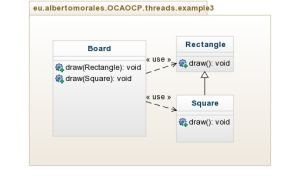Just a short post to illustrate the rules of overriding or overloading a method in Java. A deep understanding of this powerful Java mechanisms is a basic skill for every JAVA developer (as well as OOP and OOD proficiency)

Let’s start with an example.``````package eu.albertomorales.OCAOCP.threads.example3;
public class Rectangle {
public void draw() {
System.out.println("rectangle drown!");
}
}

public class Square extends Rectangle {
public void draw() {
System.out.println("square drown!");
}
}

public class Board {
public void draw(Rectangle rectangle) {
System.out.println("rectangle is going to be drown");
rectangle.draw();
}
public void draw(Square square) {
System.out.println("square is going to be drown");
square.draw();
}
public static void main(String[] args) {
Board board = new Board();
Square square = new Square();
board.draw(square);
}
}
``````

Class Board has two methods with the same name (draw) but with different argument list, which means the method is overloaded. One of them takes a Rectangle and the other one takes a Square.

If you pass a Square

``````public static void main(String[] args) {
Board board = new Board();
Square square = new Square();
board.draw(square);
}
``````

the overloaded method of class Board that takes a Square will be invoked.

Notice that Square class has an overridden method draw(). And remember, which overridden method to call is decided at runtime based on object type, so the output is what you expect:

square is going to be drown
square drown!

Lets play with polymorphism

We can create an instance of Square, and reference it as a Rectangle, because a Square object is still a Rectangle. What happens if you pass an Rectangle reference to a Square object, as an argument to the method draw() of the class Board?

``````public static void main(String[] args) {
Board board = new Board();
Rectangle rectangle = new Square();
board.draw(rectangle);
}
``````

The output now has changed:

rectangle is going to be drown
square drown!

If you pass a Rectangle reference, the overloaded method of Board that takes a Rectangle *will be invoked, even if the effective object being passed is a *Square.